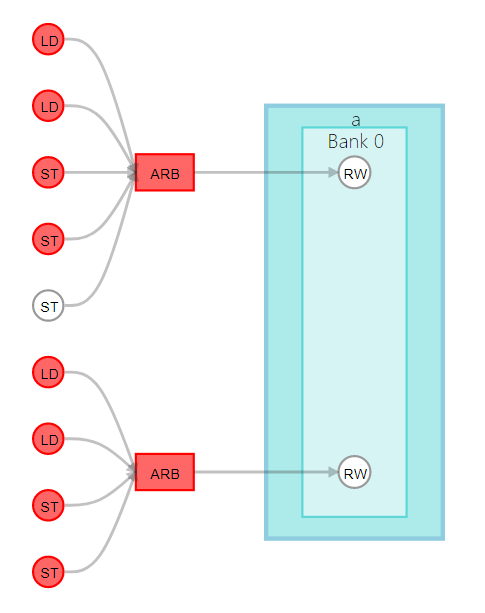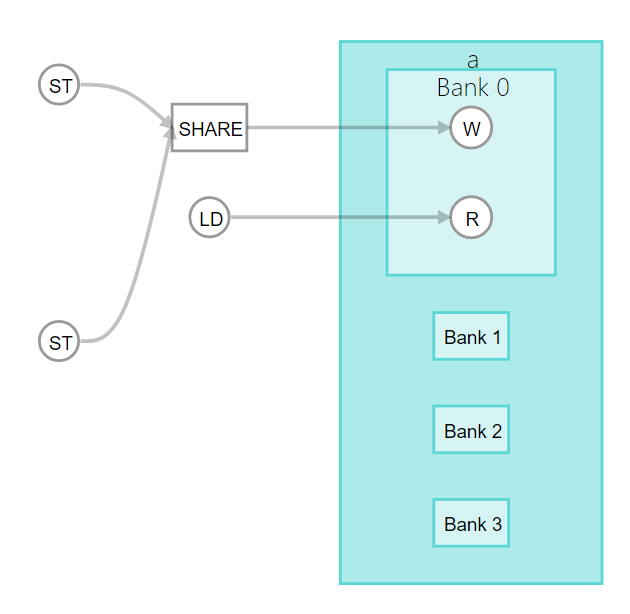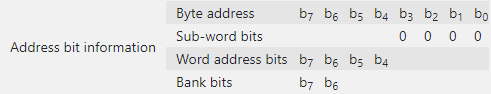ID 683152
Date 12/19/2022
Public

## 7.4. Example: Specifying Bank-Selection Bits for Local Memory Addresses

You have the option to tell the Intel® HLS Compiler Pro Edition which bits in a local memory address select a memory bank and which bits select a word in that bank. You can specify the bank-selection bits with the hls_bankbits(b 0, b 1, ..., b n) attribute.

The (b 0 , b 1 , ... ,b n ) arguments refer to the local memory address bit positions that the Intel® HLS Compiler Pro Edition should use for the bank-selection bits. Specifying the hls_bankbits(b 0, b 1 , ..., b n) attribute implies that the number of banks equals 2 number of bank bits .

 Bank 0 Bank 1 Bank 2 Bank 3 Word 0 00 000 01 000 10 000 11 000 Word 1 00 001 01 001 10 001 11 001 Word 2 00 010 01 010 10 010 11 010 Word 3 00 011 01 011 10 011 11 011 Word 4 00 100 01 100 10 100 11 100 Word 5 00 101 01 101 10 101 11 101 Word 6 00 110 01 110 10 110 11 110 Word 7 00 111 01 111 10 111 11 111
Restriction: Currently, the hls_bankbits(b 0, b 1, ..., b n) attribute supports only consecutive bank bits.

### Example of Implementing the hls_bankbits Attribute

Consider the following example component code:
1
component int bank_arbitration (int raddr,
int wdata) {

#define DIM_SIZE 4

// Adjust memory geometry by preventing coalescing
hls_numbanks(1)
hls_bankwidth(sizeof(int)*DIM_SIZE)

// Force each memory bank to have 2 ports for read/write
hls_singlepump
hls_max_replicates(1)

int a[DIM_SIZE][DIM_SIZE][DIM_SIZE];
// initialize array a…
int result = 0;

#pragma unroll
for (int dim1 = 0; dim1 < DIM_SIZE; dim1++)
#pragma unroll
for (int dim3 = 0; dim3 < DIM_SIZE; dim3++)

#pragma unroll
for (int dim1 = 0; dim1 < DIM_SIZE; dim1++)
#pragma unroll
for (int dim3 = 0; dim3 < DIM_SIZE; dim3++)

return result;
}


As illustrated in the following figure, this code example generates multiple load and store instructions, and therefore multiple load/store units (LSUs) in the hardware. If the memory system is not split into multiple banks, there are fewer ports than memory access instructions, leading to arbitrated accesses. This arbitration results in a high loop initiation interval (II) value. Avoid arbitration whenever possible because it increases the FPGA area utilization of your component and impairs the performance of your component.

Figure 41. Accesses to Local Memory a for Component bank_arbitrationBy default, the Intel® HLS Compiler Pro Edition splits the memory into banks if it determines that the split is beneficial to the performance of your component. The compiler checks if any bits remain constant between accesses, and automatically infers bank-selection bits.

Now, consider the following component code:
component int bank_no_arbitration (int raddr,
int wdata) {
#define DIM_SIZE 4

// Adjust memory geometry by preventing coalescing and splitting memory
hls_bankbits(4, 5)
hls_bankwidth(sizeof(int)*DIM_SIZE)

// Force each memory bank to have 2 ports for read/write
hls_singlepump
hls_max_replicates(1)

int a[DIM_SIZE][DIM_SIZE][DIM_SIZE];

// initialize array a…
int result = 0;

#pragma unroll
for (int dim1 = 0; dim1 < DIM_SIZE; dim1++)
#pragma unroll
for (int dim3 = 0; dim3 < DIM_SIZE; dim3++)

#pragma unroll
for (int dim1 = 0; dim1 < DIM_SIZE; dim1++)
#pragma unroll
for (int dim3 = 0; dim3 < DIM_SIZE; dim3++)

return result;
}

The following diagram shows that this example code creates a memory configuration with four banks. Using bits 4 and 5 as bank selection bits ensures that each load/store access is directed to its own memory bank.

Figure 42. Accesses to Local Memory a for Component bank_no_arbitrationIn this code example, setting hls_numbanks(4) instead of hls_bankbits(4,5) results in the same memory configuration because the Intel® HLS Compiler Pro Edition automatically infers the optimal bank selection bits.

In the Function Memory Viewer (inf the High-Level Design Reports), the Address bit information shows the bank selection bits as b6 and b7, instead of b4 and b5:This difference occurs because the address bits reported in the Function Memory Viewer are based on byte addresses and not element addresses. Because every element in array a is four bytes in size, bits b4 and b5 in element address bits correspond to bits b6 and b7 in byte addressing.

1 For this example, the initial component was generated with the hls_numbanks attribute set to 1 (hls_numbanks(1)) to prevent the compiler from automatically splitting the memory into banks.